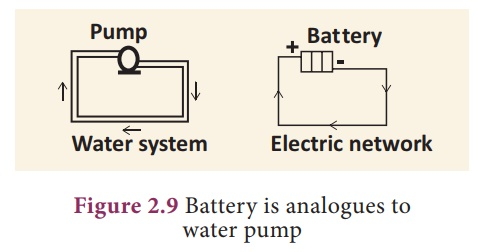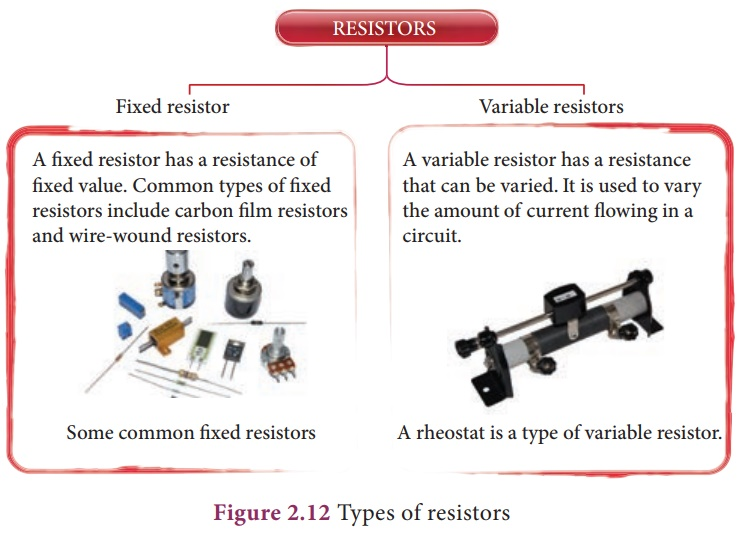Home | | Science 9th std | Electric current

# Electric current

When the charged object is provided with a conducting path, electrons start to flow through the path from higher potential to lower potential region.

Electric current

When the charged object is provided with a conducting path, electrons start to flow through the path from higher potential to lower potential region. Normally, the potential difference is produced by a cell or battery. When the electrons move, we say that an electric current is produced. at is, an electric current is formed by moving electrons.

## 1. Direction of current

Before the discovery of the electrons, scientists believed that an electric current consisted of moving positive charges. Although we know this is wrong, the idea is still widely held, as the discovery of the flow of electrons did not a ect the basic understanding of the electric current. The movement of the positive charge is called as ‘conventional current’. The flow of electrons is termed as ‘electron current’. is is depicted in Figure 2.6.In a battery, the potential of the positive terminal is maintained positive and the negative terminal is negative. Electrons are removed from the positive terminal and enriched at the negative terminal internally by means of chemical reaction or other processes. When a connection is given externally by a conducting wire, electrons flow from the negative terminal to the positive of the cell. Conventional current or simply the current, behaves as if positive charges cause the current ow. Although in reality it is the electron that moves in one direction, in equivalence, we consider as if it is the positive charges are moving in the opposite direction. is is taken as the direction of ‘current’.

In electrical circuits the positive terminal is represented by a long line and negative terminal as a short line. Battery is the combination of more than one cell as shown in the Fig. 2.7.## 2. Measurement of electriccurrent

We can measure the value of current and express it numerically. Current is the rate at which charges flow past a point on a circuit.

That is, if q is the quantity of charge passing through a cross section of a wire in a time t, quantity of current (I) is represented as,

I = q/t

The standard SI unit for current is ampere with the symbol A. Current of 1 ampere means that there is one coulomb (1C) of charge passing through a cross section of a wire every one second (1 s).

1 ampere = 1 coulomb / 1 second (or)

1 A = 1 C / 1 s = 1CS-1

Ammeter is an instrument used to measure the strength of the electric current in an electric circuit.The ammeter is connected in series in a circuit where the current is to be found. . The current flows through the positive ‘+’ red terminal of ammeter and leaves from the negative ‘–’ black terminal.

Exercise 2.2

Suppose, 25 C of charge is determined to pass through a wire of any cross section in 50 s, what is the measure of current?

### Solution:

I = q / t = (25 C) / (50 s) = 0.5 C/s = 0.5 A

### Exercise 2.3

The current flowing through a lamp is 0.2A. If the lamp is switched on for one hour, What is the total electric charge that passes through the lamp?

### Solution:

I = q / t; q = I x t

Time has to be in second.

1hr = 1x 60 x 60 s= 3600 s

q = I x t = 0.2A x 3600s = 720C

## 3. Electromotive force (e.m.f)

Imagine that two ends of a water pipe lled with water are connected. Although lled with water, the water will not move or circle around the tube on its own. Suppose, you insert a pump in between and the pump pushes the water, then the water will start moving in the tube. Now the moving water can be used to produce some work. We can insert a water wheel in between the flow and make it to rotate and further use that rotation to operate machinery.

Likewise if you take a circular copper wire, it is full of free electrons. However, they are not moving in a particular direction. You need some force to push the electrons to move in a direction. The water pump and a battery are compared in Figure 2.9.Devices like electric cells and other electrical energy sources act like pump, ‘pushing’ the charges to flow through a wire or conductor. The ‘pumping’ action of the electrical energy source is made possible by the ‘electromotive force (e.m.f). The electromotive force is represented as (e). The e.m.f of an electrical energy source is the work done (W) by the source in driving a unit charge (q) around the complete circuit.

ε = W/q

where, W is the work done or the non-electrical energy converted into electrical energy measured in joules and q is the amount of charge. The SI unit of e.m.f is joules per coulomb (JC-1) or volt (V). In other words the e.m.f of an electrical energy source is one volt if one joule of work is done by the source to drive one coulomb of charge completely around the circuit.

### Exercise 2.4

The e.m.f of a cell is 1.5V. What is the energy provided by the cell to drive 0.5 C of charge around the circuit?

Solution: ε = 1.5V and q = 0.5C

ε = W/q; W= ε x q; Therefore W = 1.5 x 0.5 = 0.75J

## 4. Potential difference (p.d)

One does not just let the circuit connect one terminal of a cell to another. O en we connect, say a bulb or a small fan or any other electrical device in an electric circuit and use the electric current to drive them. is is how a certain amount of electrical energy provided by the cell or any other source of electrical energy is converted into other form of energy like light, heat, mechanical and so on. For each coulomb of charge passing through the light bulb (or any appliances) the amount of electrical energy converted to other forms of energy depends on the potential difference across the electrical device or any electrical component in the circuit. The potential difference is represented by the symbol V.

V = W/q

where, W is the work done, that is the amount of electrical energy converted into other forms of energy measured in joule and q is amount of charge measured in coulomb. The SI unit for both e.m.f and potential difference is the same in volt (V).

Voltmeter is an instrument used to measure the potential difference. To measure the potential difference across a component in a circuit, the voltmeter must be connected in parallel to it. Say, you want to measure the potential difference across a light bulb you need to connect the voltmeter as given in Figure 2.10.Note the positive (‘+’) red terminal of the voltmeter is connected to the positive side of circuit and the negative (‘–’) black terminal is connected to the negative side of the circuit across a component (light bulb in the above illustration).

### Exercise 2.5

A charge of 2x104 C flows through an electric heater. The amount of electrical energy converted into thermal energy is 5 MJ. Compute the potential difference across the ends of the heater.

V = W/q that is 5x106 J / 2x104

C = 250 V

## 5. Resistance

The Resistance (R) is the measure of opposition o ered by the component to the flow of electric current through it. The opposition to the flow of current is caused in terms of opposition to the flow of electrons by other electrons and the thermal vibrations. Different electrical components o er different electrical resistance.

Even the conducting wires o er resistance to the flow of electric current through it. But, it is very much negligible. Metals like copper, aluminium etc., have very much negligible resistance. at is why they are called good conductors. On the other hand, materials like nicrome, tin oxide etc., o er high resistance to the electric current. We also have a category of materials called insulators; they do not conduct electric current at all (Glass, Polymer, rubber and paper). All these materials are needed in electrical circuits to have usefulness and safety in electrical circuits.

The resistance offered by a material at a particular temperature depends on the,

i. geometry of the material and

ii. nature of the material.

The SI unit of resistance is ohm with the symbol (Ω). One ohm is the resistance of a component when the potential difference of one volt applied across the component drives a current of one ampere through it.

We can also control the amount of flow of current in a circuit with the help of resistance. Such components used for providing resistance are called as ‘resistors’. The resistors can be xed or variable.Fixed resistors have xed value of resistance, while the variable resistors like rheostats can be used to obtain desired value of resistance as shown in Figure 2.11.

## 6. Ohm’s law

Ohm’s law states that electric potential difference across two points in an electrical circuit is directly proportional to the current passing through it. That is,

V ∞ I

The proportionality constant is the resistance (R) offered between the two points.

Hence, Ohm’s law is written as,

V = R I (or) V = I R

Where V is the potential difference across the component in volt(V), I is the current flowing through the component in ampere(A) and R is the resistance of the component in ohm ( ).

Any appliance connected to the circuit o ers resistance. We can measure it by measuring the current (I) owing through them and the potential difference (V) across them. Once we measure these two quantities, we can compute R from the formula R=V/I. When we plot a graph by taking current (I) in the x-axis and voltage (V) in the y-axis, we get a straight line as shown in Fig 2.13. The slope of the line gives the value of resistance (R)### Example 2.6

A potential difference of 230 V applied across the heating coil drives a current of 10 A through it. Calculate the resistance of the coil.

V = 230 V; I = 10A

R = V/ I that is 230/10 = 23

Study Material, Lecturing Notes, Assignment, Reference, Wiki description explanation, brief detail
9th Science : Electric charge and electric current : Electric current |